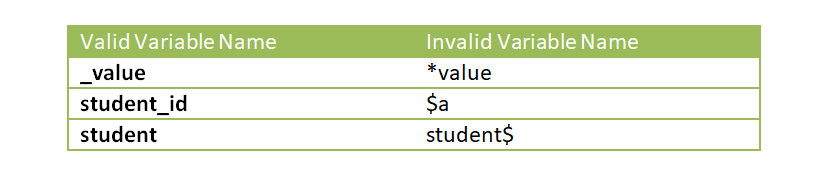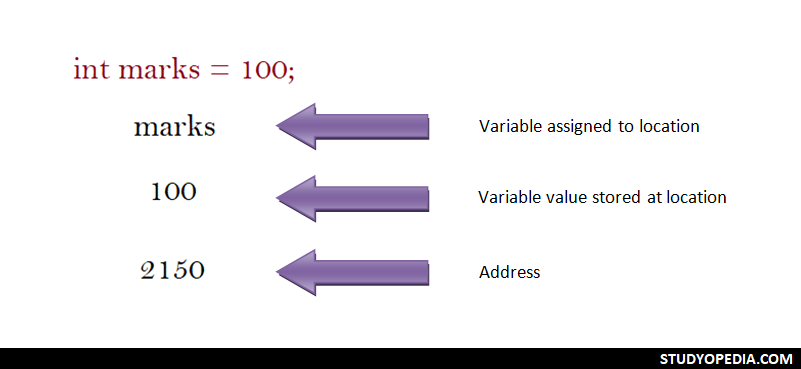## 21 Jan C++ Variables

Variable names in C++ are the names given to locations in memory. These locations can be an integer, characters, or real constants. In addition, a variable type can only hold the same constant type. In this lesson, we will learn how to work with Variables in C++.

## Rules for naming a variable in C++

The following are the rules for naming a variable: The first character in a variable name must be an underscore or alphabet. Here are the rules, with some examples,

• A variable name is a combination of alphabets 1 to 31, digits, or underscores.• No special symbol in a variable name is valid, except the underscore.• No comma is allowed in a variable name.
• In addition, no blank space is valid.

Always try to create meaningful names. For example, the variable names for calculating the results of students can be given variable name results.

## Variable Declaration

Declaration of a variable in C++ is quite easy. The following is the syntax showing how to declare variables in C++,

The valid variable declaration examples are shown below. Here, intchar, and float are datatypes,

The preceding examples show how a variable is declared, wherein a datatype precedes the variable name.

The following examples demonstrate the usage of variables in C++,[email protected]

We work to create programming tutorials for all.